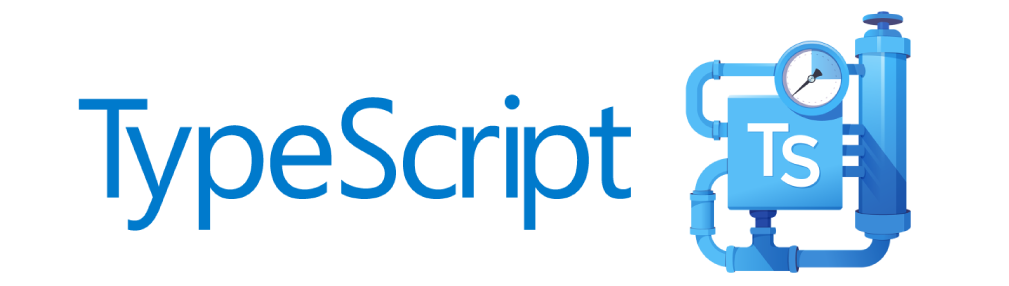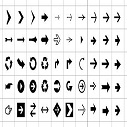# Hackerrank - Cavity Map Problem

You are given a square map as a matrix of integer strings. Each cell of the map has a value denoting its depth. We will call a cell of the map a cavity if and only if this cell is not on the border of the map and each cell adjacent to it has strictly smaller depth. Two cells are adjacent if they have a common side, or edge.

Find all the cavities on the map and replace their depths with the uppercase character X.

For example, given a matrix:

989
191
111


You should return:

989
1X1
111


The center cell was deeper than those on its edges: [8,1,1,1]. The deep cells in the top two corners don't share an edge with the center cell.

Function Description

Complete the cavityMap function in the editor below. It should return an array of strings, each representing a line of the completed map.

cavityMap has the following parameter(s):

• grid: an array of strings, each representing a row of the grid

Input Format

The first line contains an integer , the number of rows and columns in the map.

Each of the following lines (rows) contains positive digits without spaces (columns) representing depth at .

Constraints

Output Format

Output lines, denoting the resulting map. Each cavity should be replaced with the character X.

Sample Input

4
1112
1912
1892
1234


Sample Output

1112
1X12
18X2
1234


Explanation

The two cells with the depth of 9 are not on the border and are surrounded on all sides by shallower cells. Their values have been replaced by X.

### Solution

#!/bin/python3

import math
import os
import random
import re
import sys

# Complete the cavityMap function below.
def cavityMap(grid):
v = []
for i, v in enumerate(grid[1:-1], 1):
for x, j in enumerate(v[1:-1], 1):
m = j
if m > v[x - 1] and m > v[x + 1] and m > grid[i - 1][x] and m > grid[i + 1][x]:
v = v[:x] + 'X' + v[x + 1:len(v)]
grid[i] = v
return grid

if __name__ == '__main__':
fptr = open(os.environ['OUTPUT_PATH'], 'w')

n = int(input())

grid = []

for _ in range(n):
grid_item = input()
grid.append(grid_item)

result = cavityMap(grid)

fptr.write('\n'.join(result))
fptr.write('\n')

fptr.close()Akd on Jul 18, 2020 at 06:07 am

#### Post Comment

##### WEB TECHNOLOGY##### TypeScript Programmning Language##### New HTML5 APIs##### HTML Arrow -> Range: Decimal 8592-8703. Hex 2190-21FF.##### How To Override One CSS Class With Another×